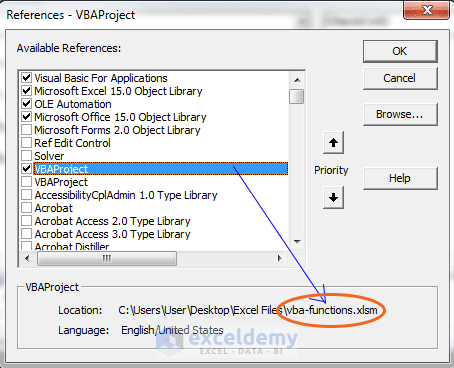Disclosure: This post may contain affiliate links, meaning when you click the links and make a purchase, we receive a commission.

# How to Execute a Function Procedure

We know how to create macro Sub procedure. Macro Sub procedure can be executed in many ways. But, Function procedure can be executed only in two ways:

• Function procedure can be called from another VBA procedure.
• You can use it a worksheet formula like predefined Excel functions.

## Calling custom functions from a procedure

Custom functions can be called from another VBA procedure (VBA functions or VBA Sub procedure whatever it is).

Say you have designed a function called CalculateTax (Amount, TaxRate). This function takes Amount and TaxRate from the user and returns the calculated tax for the user. We can use this function like the following statement:

`Tax = CalculateTax(Amount, TaxRate)`

Whatever the amount this function returns, is assigned to the Tax variable.

## Using custom functions in a worksheet formula

We use a custom function in a worksheet formula just like we use predefined Excel functions. However, you have to make sure that Excel can locate your custom function.

There exist two cases: your function procedure can be in the same workbook or in the different workbook. If the function procedure is in the same workbook, you don’t need to do anything to locate the function for Excel. If the function is defined in a different workbook, you may have to instruct Excel where to find the function.

Read More: A detailed analysis of Excel VBA Function Procedure Arguments

You can do this in the following three ways:

### Precede the function’s name with a file reference.

For example, say you want to use function NumberSign(Number) function and it is defined in vba-functions.xlsm workbook. You want to use NumberSign(Number) function in Number-check.xlsm workbook (now you’re working with this file). You can use a reference such as the following:
=’vba-functions.xlsm’!NumberSign(A3). vba-functions.xlsm workbook must be open when you’re writing references for Number-check.xlsm workbook.

See the image below for a clear idea.Referencing workbook to use its defined function.

If you insert the function with the Insert Function dialog box, the workbook reference is inserted automatically. To activate Insert Function dialog box choose Formulas ➪ Function Library ➪ Insert Function Wizard.

## Set up a reference to the workbook

You can create references to the workbook where the custom function is defined. In this process, you don’t have to precede the custom function name with the workbook name. In the VB Editor, choose Tools ➪ References to establish a reference to another workbook.

See the following figure. A list of references is presented including all open workbooks. Place a checkmark in the item that refers to the workbook that contains the custom function. If the workbook isn’t open, click Browse to choose the workbook.Select the workbook where the custom function is defined to create references.